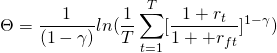# Manipulation Proof Performance Measure (MPPM)

The Manipulation Proof Performance Measure (MPPM) is a performance measure introduced by Goetzmann, Ingersoll, Spiegel and Welch in 2007 called “Portfolio Performance Manipulation and Manipulation-proof Performance Measures”. Unlike a number of other measures, the MPPM is defined in such a way that it is hard for fund managers to game the performance measure.

On this page, we discuss the manipulation proof performance measure definition, provide the formula and discuss when the measure is most commonly uses.

## Manipulation Proof Performance Measure definition

The Manipulation Proof Performance Measure was introduced to address an important issue with existing performance measures. While most performance measures try to capture the enhancements of active management as well as possible, there is clearly be a problem. In particular, the portfolio managers know which measure are used to assess their performance. Thus, they have an incentive to manipulate their performance such that they perform well based on the performance measure.

The work of Goetzmann, Ingersoll, Spiegel, and Welch discusses the likely impact that this gaming may have on existing performance measures. They find that most performance measures can be gamed, even when transaction costs are really high. Thus, given this observation, they propose a new measure, the MPPM .

The MPPM is an average of a power utility function, calculated over the return horizon. Next, let’s look at the manipulation proof performance measure formula.

## Manipulation Proof Performance Measure  formula

The MPPM formula is the followingwhere T is the number of observations, gamma is a parameter related to risk aversion, rft is the risk-free rate, deltat is the length of the period in years, rt is the return of the fund.

## When to use the MPPM?

The use of the manipulation proof performance metric is especially useful in the context of hedge funds. These funds have access to derivatives and their manager compensation makes them very sensitive to potential opportunities to manipulate their performance.

## Summary

The MPPM is a fairly new performance measure which is used commonly in the hedge fund industry to assess performance. It can easily be calculate in an Excel spreadsheet using fund return data.